# Kinematics Quiz 1

#### Quiz Description

In this quiz, we shall study one important aspect of physics known as kinematics. We shall look at velocity, speed, and velocity - time graphs, acceleration, etc. Graphs are something very common you would find in this topic, implying that knowing it to your fingertips is a plus for you. Answering the quiz will give you an upper hand in the understanding of kinematics as a whole.

Kinematics is a branch of mechanics that studies the motion of a point, body, or group of objects, without considering the cause of the motion. This topic greatly focuses on aspects like acceleration and velocity. To describe the motion of celestial bodies, astrophysicists make use of kinematics. Kinematics could also be applied in biomechanics by describing the motion of the skeletal system.

Now we have come to realize how important this quiz is to anyone studying kinematics. Are you looking for a way of understanding this topic? All you need to do is take the A level physics quiz, and challenge yourself. Good luck!

The graph shows how the speed of a car varies with time. Which statement about the acceleration of the car between 10 s and 20 s is correct?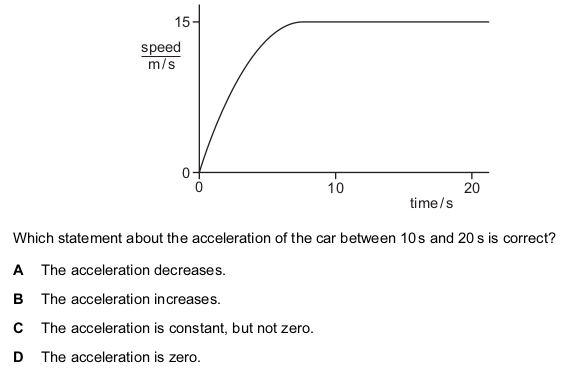Correct
• A
• B
• C
• D

A student walks at a constant speed. He takes 100 s to walk 160 paces. The length of each pace is 0.80 m.

How far does the student walk in 50 s?

Correct
• 64m
• 80m
• 128m
• 256m

A student drops, from rest, a table-tennis ball in air.

What happens to the velocity and to the acceleration of the ball during the first few seconds after release?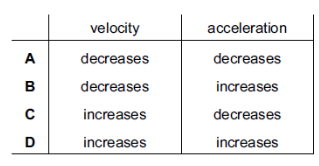Correct
• A
• B
• C
• D

A cyclist takes a ride lasting 25 s.

The diagram shows how her distance traveled from the starting position varies with time.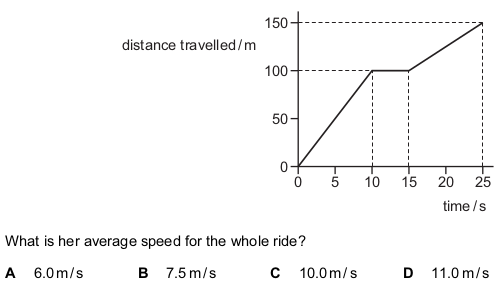Correct
• A
• B
• C
• D

A car begins to move. It speeds up until it reaches a constant speed. It continues to travel at this constant speed for the rest of the journey.

What happens to the acceleration and what happens to the velocity of the car during the journey?

Correct
• Both the acceleration and the velocity change.
• Only the acceleration changes.
• Only the velocity changes.
• Neither the acceleration nor the velocity changes.

An object travels at a constant speed of 10 m / s for 10 s. During the next 5 s, it accelerates uniformly to 20 m / s.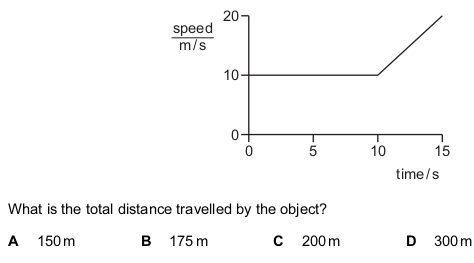Correct
• A
• B
• C
• D

A lorry takes 15 minutes to travel along the path PQRS.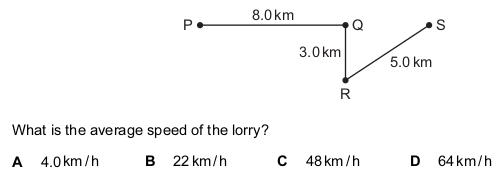Correct
• A
• B
• C
• D

At time t 1 , a stone is dropped from a stationary balloon. The stone reaches terminal velocity at time t 2 .

Which row gives the acceleration of the stone at time t 1 and at time t 2 ?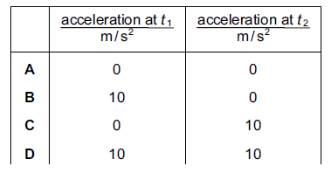Correct
• A
• B
• C
• D

The graph shows how the speed of a car travelling in a straight line changes with time.

Which section shows the largest acceleration?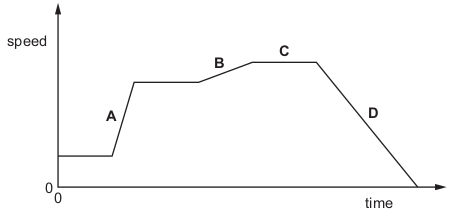Correct
• A
• B
• C
• D

An object falls from rest through the air and the air resistance on it increases. The object reaches terminal velocity.

Which quantity decreases until its terminal velocity is reached?

Correct
• acceleration
• kinetic energy
• speed
• weight

An object falls from rest through the air. Its velocity increases until it reaches terminal velocity.

Which quantity increases until its terminal velocity is reached?

Correct
• acceleration
• air resistance
• resultant force
• weight

The speed-time graph represents a short journey.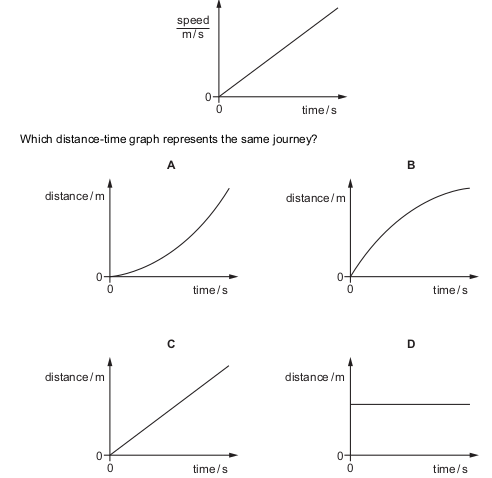Correct
• A
• B
• C
• D

When a heavy coin falls a short distance towards the ground it does not reach terminal velocity.

Why is this?

Correct
• The coin has not hit the ground.
• The weight of the coin equals the air resistance.
• The weight of the coin increases as air resistance increases.
• The weight of the coin is always more than air resistance.

A car travels along a road at 50 km / h. The driver applies the same braking force at the same place on a day when the surface is dry

and then on a day when the road is wet. On the wet surface, how many of these distances are greater than on the dry surface?

braking distance

stopping distance

thinking distance

Correct
• 0
• 1
• 2
• 3

The speed-time graph for a falling skydiver is shown below. As he falls, the skydiver spreads out his arms and legs and then opens his parachute.

Which part of the graph shows the skydiver falling with terminal velocity?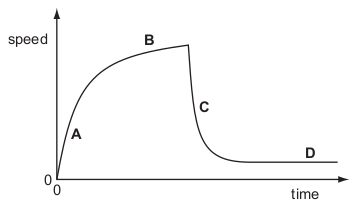Correct
• A
• B
• C
• D

An object moves from P to Q in 10 s with uniform acceleration.

velocity at P = 5 m / s

velocity at Q = 12 m / s

What is the acceleration?

Correct
• 0.5 m / s 2
• 0.5 m / s 2
• 1.2 m / s 2
• 1.2 m / s 2

The graph shows how the height of an object above the ground changes with time.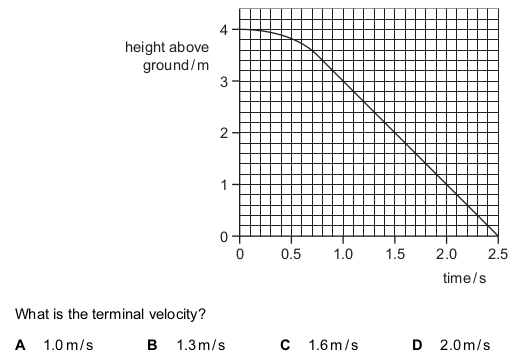Correct
• A
• B
• C
• D

The speed-time graph represents the journey of a car.

The dots separate different sections of the journey. There are six different sections.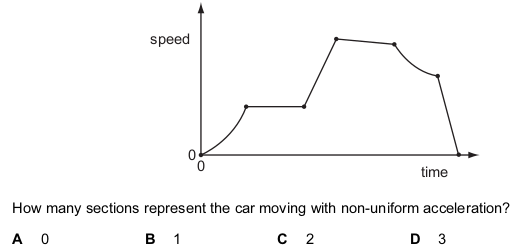Correct
• A
• B
• C
• D

A steel ball is released just below the surface of thick oil in a cylinder.

During the first few centimetres of travel, what is the acceleration of the ball?

Correct
• constant and equal to 10 m / s 2
• constant but less than 10 m / s 2
• decreasing
• increasing

The diagram shows the speed-time graph of the motion of a car for four seconds.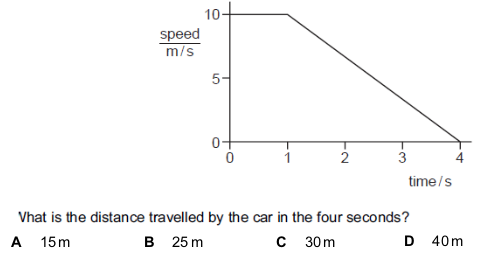Correct
• A
• B
• C
• D